# Trigonometry : Graphing Sine and Cosine

## Example Questions

### Example Question #1 : Graphing Sine And Cosine

The function shown below has an amplitude of ___________ and a period of _________.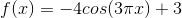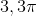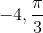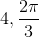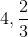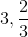Explanation:

The amplitude is always a positive number and is given by the number in front of the trigonometric function.  In this case, the amplitude is 4.  The period is given by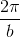, where b is the number in front of x.  In this case, the period is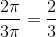.

### Example Question #1 : Graphing Sine And Cosine

This is the graph of what function?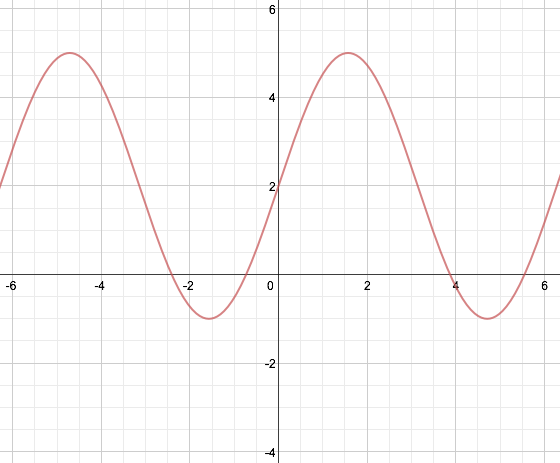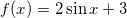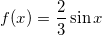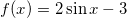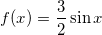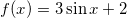Explanation:

The amplitude of the sine function is increased by 3, so this is the coefficient for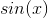. The +2 shows that the origin of the function is now at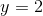instead of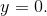### Example Question #1 : Graphing Sine And Cosine

Which of the following graphs does not have a-intercept at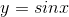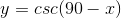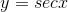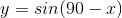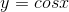Explanation:

The y-intercept is the value of y when.

Recall that cosine is thevalue of the unit circle. Thus,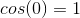, so it works.

Secant is the reciprocal of cosine, so it also works.

Also recall that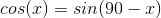. Thus, the only answer which is not equivalent is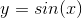### Example Question #1 : Graphing Sine And Cosine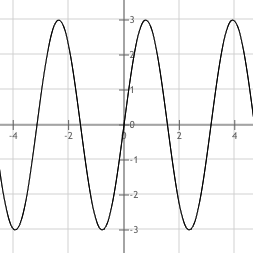What is an equation for the above function?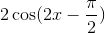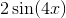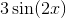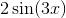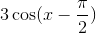Explanation:

The amplitude of a sinusoidal function isunless amplified by a constant in front of the equation. In this case, the amplitude is, so the front constant is.

The graph moves through the origin, so it is either a sine or a shifted cosine graph.

It repeats once in every, as opposed to the usual, so the period is doubled, the constant next to the variable is.

The only answer in which both the correct amplitude and period is found is:### Example Question #51 : Trigonometric Functions And Graphs

Let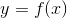be a function defined as follows: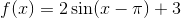.

The 3 in the function above affects what attribute of the graph of?

Phase shift

Period

Amplitude

Vertical shift

Vertical shift

Explanation:

The period of the function is indicated by the coefficient in front of; here the period is unchanged.

The amplitude of the function is given by the coefficient in front of the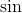; here the amplitude is 2.

The phase shift is given by the value being added or subtracted inside thefunction; here the shift isunits to the right.

The only unexamined attribute of the graph is the vertical shift, so 3 is the vertical shift of the graph.

### Example Question #1 : Graphing Sine And Cosine

Which graph correctly illustrates the given equation?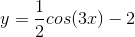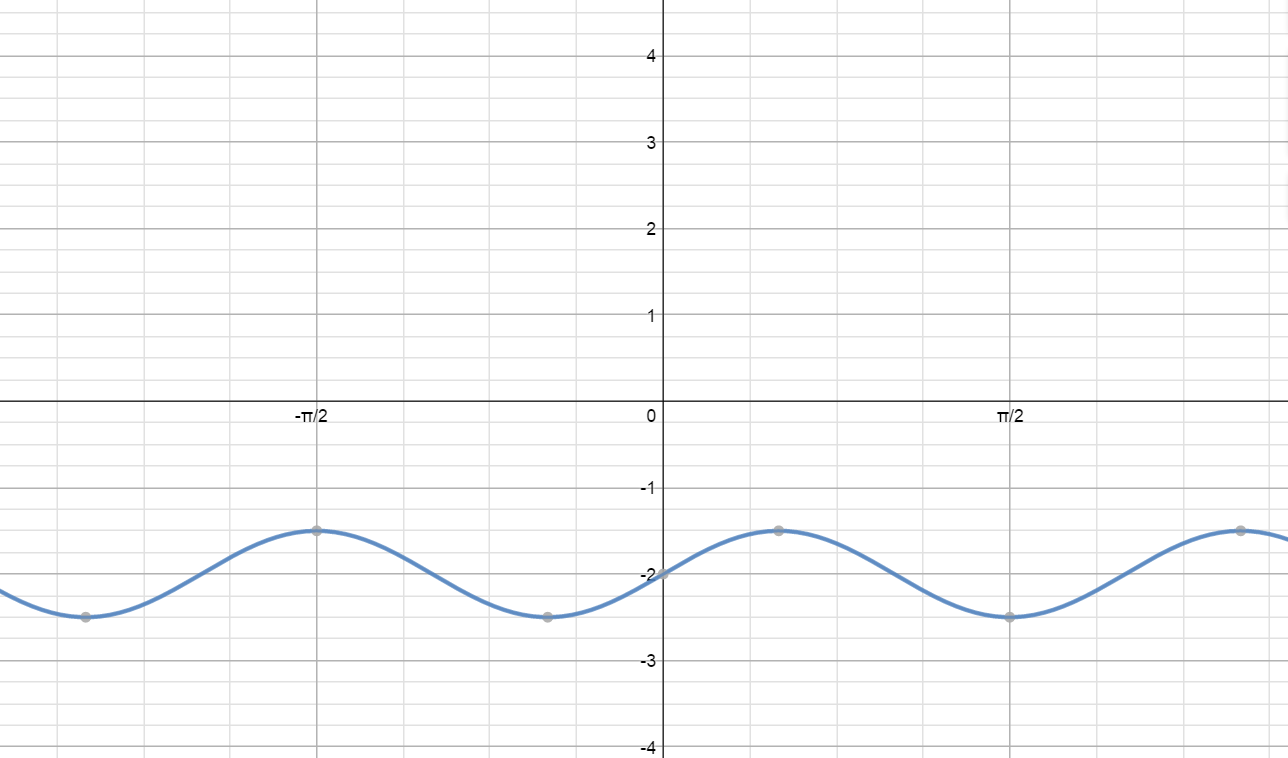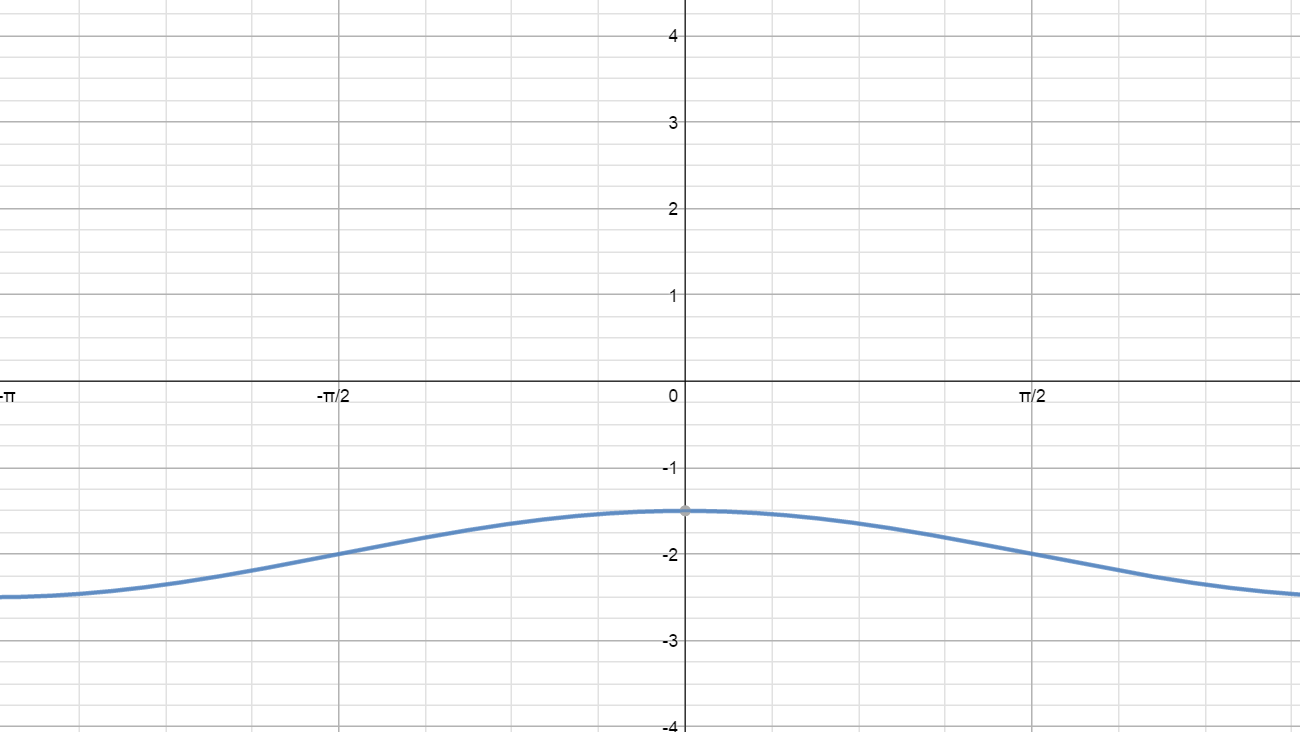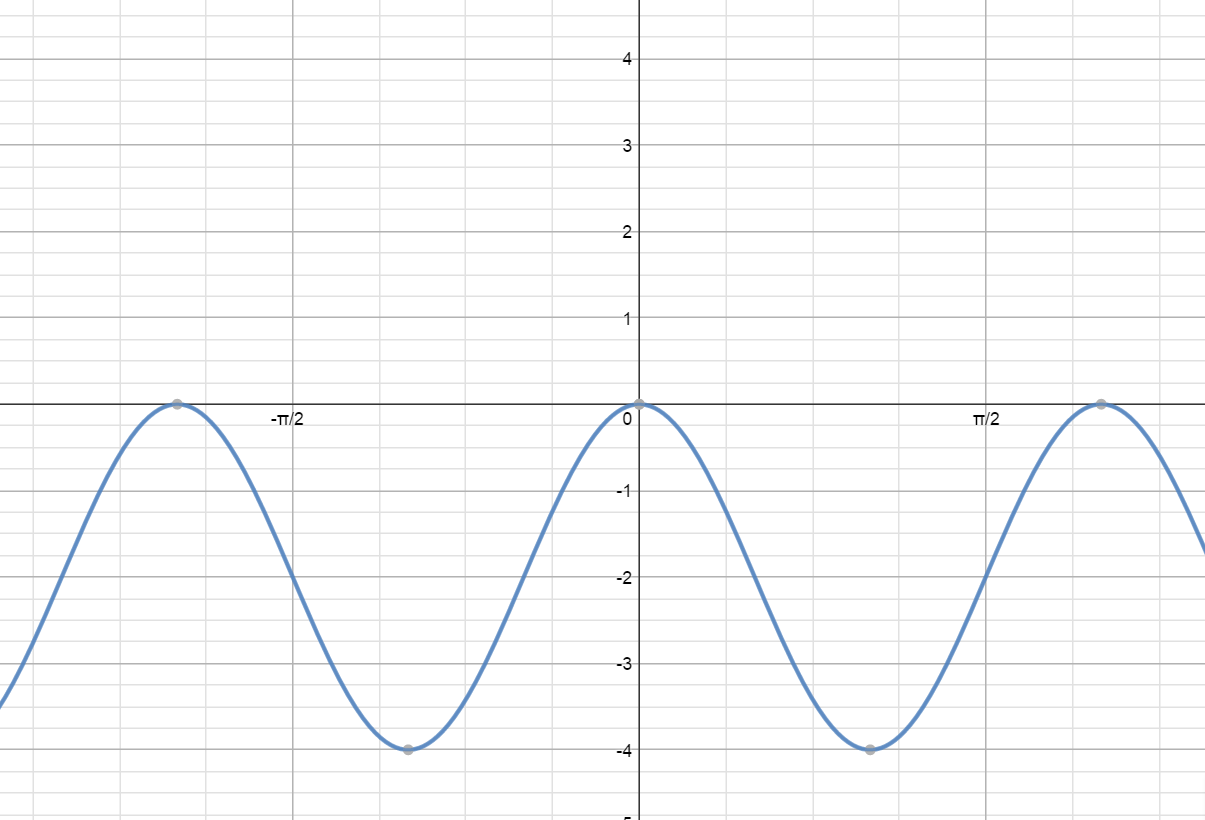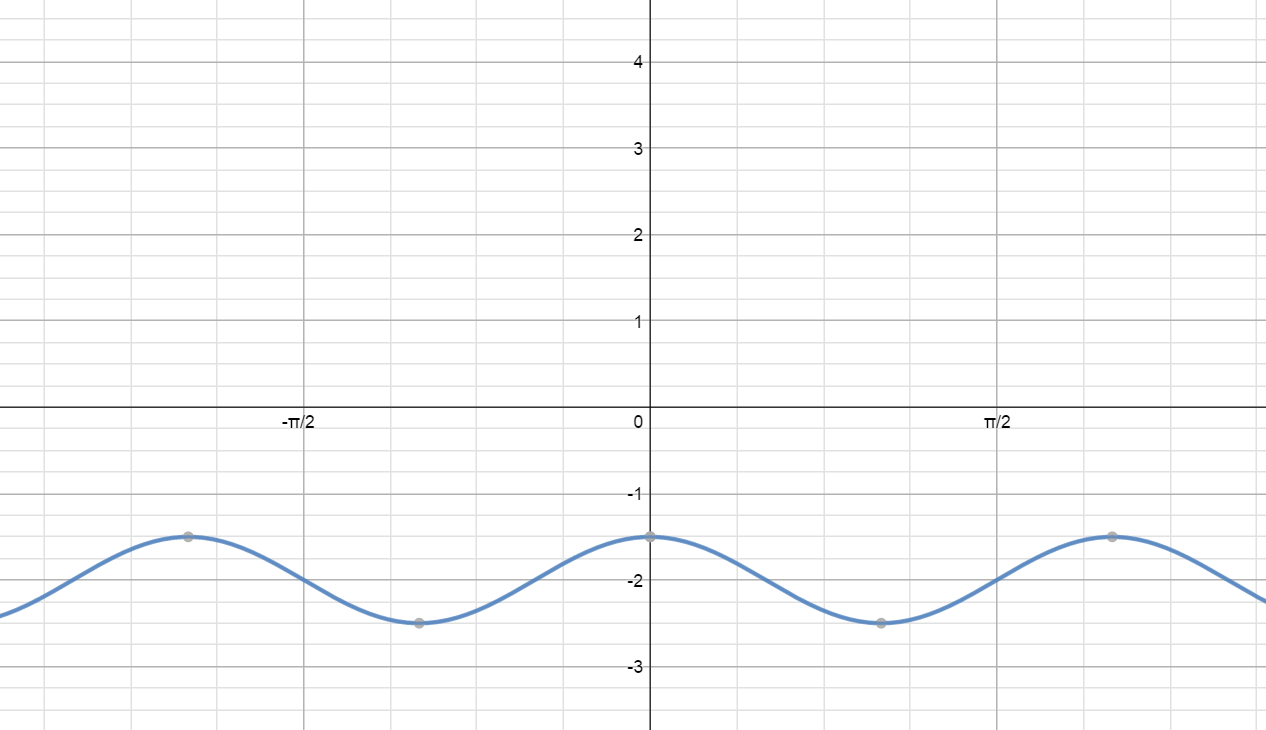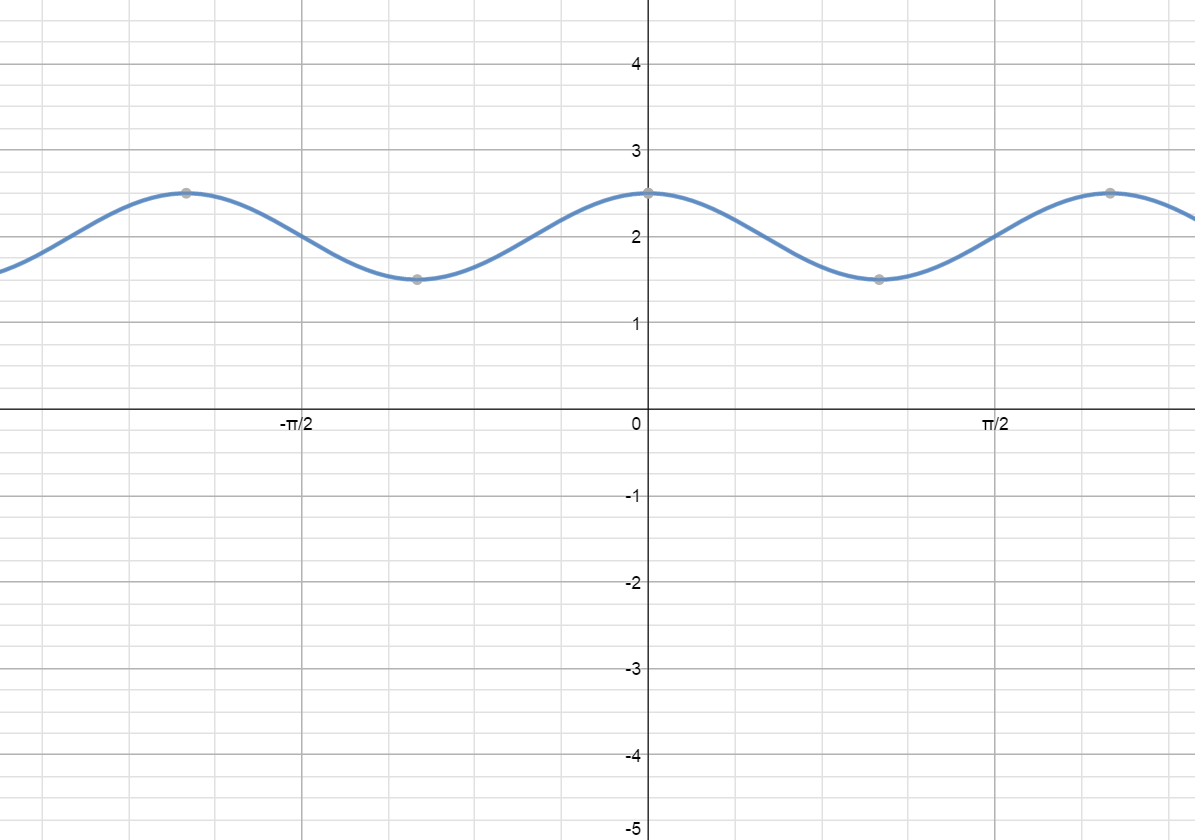Explanation:

The simplest way to solve a problem like this is to determine where a particular point on the graph would lie and then compare that to our answer choices. We should first find the y-value when the x-value is equal to zero. We will start by substituting zero in for the x-variable in our equation.Now that we have calculated the y-value we know that the correct graph must have the following point:Unfortunately, two of our graph choices include this point; thus, we need to pick a second point.

Let's find the y-value when the x-variable equals the following: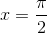We will begin by substituting this into our original equation.Now we need to investigate the two remaining choices for the following point:Unfortunately, both of our remaining graphs have this point as well; therefore, we need to pick another x-value. Suppose the x-variable equals the following: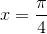Now, we must substitute this value into our given equation.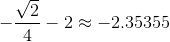Now, we can look for the graph with the following point:We have narrowed in on our final answer; thus, the following graph is correct:### All Trigonometry Resources